Haar measure

(diff) ← Older revision | Latest revision (diff) | Newer revision → (diff)

A non-zero positive measureon the-ringof subsetsof a locally compact groupgenerated by the family of all compact subsets, taking finite values on all compact subsets of, and satisfying either the condition of left-invariance: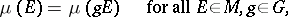where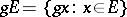, or the condition of right-invariance: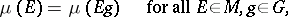where. Accordingly, one speaks of a left- or right-invariant Haar measure. Every Haar measure is-regular, that is,for all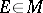.

A left-invariant (and also a right-invariant) Haar measure exists and is unique, up to a positive factor; this was established by A. Haar  (under the additional assumption that the groupis separable).

Ifis a continuous function of compact support on, thenis integrable relative to a left-invariant Haar measure on, and the corresponding integral is left-invariant (see Invariant integration), that is,for all. A right-invariant Haar measure has the analogous property. The Haar measure of the whole groupis finite if and only ifis compact.

Ifis a left-invariant Haar measure on, then for every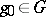the following equality holds: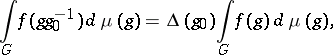whereis a continuous homomorphism ofinto the multiplicative groupof positive real numbers that does not depend on the choice of the continuous functionof compact support on. The homomorphismis called the modulus of; the measureis a right-invariant Haar measure on. If, thenis called unimodular; in this case a left-invariant Haar measure is also right-invariant and is called (two-sided) invariant. In particular, every compact or discrete or Abelian locally compact group, and also every connected semi-simple or nilpotent Lie group, is unimodular. Unimodularity of a groupis also equivalent to the fact that every left-invariant Haar measureonis also inversely invariant, that is,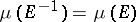for all.

Ifis a Lie group, then the integral with respect to a left-invariant (right-invariant) Haar measure onis defined by the formula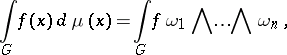where the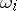are linearly independent left-invariant (right-invariant) differential forms of order one on(see Maurer–Cartan form) and. The modulus of a Lie groupis defined by the formula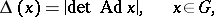where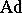is the adjoint representation (cf. Adjoint representation of a Lie group).

Examples. 1) The Haar measure on the additive groupand on the quotient group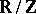(the group of rotations of the circle) is the same as the ordinary Lebesgue measure. 2) The general linear group,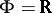or, is unimodular, and the Haar measure has the form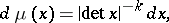where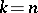forand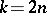for, and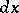is the Lebesgue measure in the Euclidean space of all matrices of orderover the field.

Ifis a locally compact group,is a closed subgroup of it,is the homogeneous space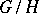,andare the moduli ofand, respectively, andis a continuous homomorphism ofinto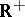whose restriction tois given by the formula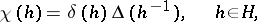then there exists a positive measureon the-ringof setsthat is generated by the family of compact subsets of; it is uniquely determined by the condition: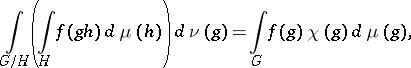whereis any continuous function of compact support on,, andfor all continuous functionsof compact support on.